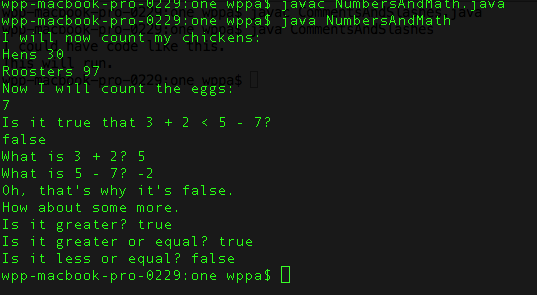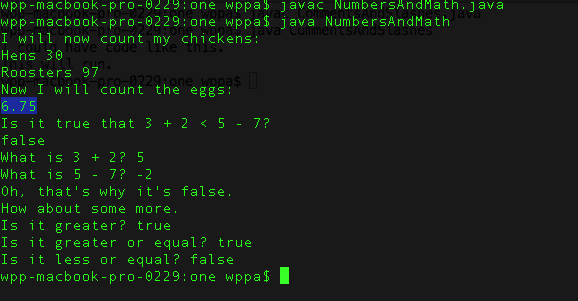# Assignment #11 and NumbersAndMath

## Code

```    /// Name: Mahima Choudhary
/// Period: 6
/// Program Name: NumbersAndMath.java
/// File Name: NumbersAndMath
/// Date Finished: 9/15/2015

public class NumbersAndMath
{
public static void main( String[] args )
{
// this is a command to write a string on console
System.out.println( "I will now count my chickens:" );

// divide 30 by 6 and add to 25 and then print it on console concatenated with String Hens
System.out.println( "Hens " + ( 25 + 30 / 6 ) );

// multiply 25 by 3 and divide by 4 to get a remainder of 3, then subtract 100 by 3
// and then print it on console concatenated with String Roosters
System.out.println( "Roosters " + ( 100 - 25 * 3 % 4 ) );

// this is a command to write a string on console
System.out.println( "Now I will count the eggs:" );

// add 3 with 2 and 1, subtract 5, add 4, divide by 2 and get remainder subtract 1, divide by 4 and add 6
//the result is 6.5 and will round to 7
System.out.println( 3 + 2 + 1 - 5 + 4 % 2 - 1 / 4 + 6 );

// this is a command to write a question string on console
System.out.println( "Is it true that 3 + 2 < 5 - 7?" );

// add 3 by 2 and subtract 5 by 7
System.out.println( 3 + 2 < 5 - 7 );

System.out.println( "What is 3 + 2? " + ( 3 + 2 ) );

//subtracting 5 by 7
System.out.println( "What is 5 - 7? " + ( 5 - 7 ) );

// this is a command to write a string on console
System.out.println( "Oh, that's why it's false." );

// this is a command to write a string on console
System.out.println( "How about some more." );

// compare the values of 5 and -2, is it greater?
System.out.println( "Is it greater? " + ( 5 > -2 ) );

//compare the values of 5 and -2, is it greater or equal?
System.out.println( "Is it greater or equal? " + ( 5 >= -2 ) );

//compare the values of 5 and -2, is it equal?
System.out.println( "Is it less or equal? " + ( 5 <= -2 ) );
}
}
```

### Picture of the output## Variation with Floating Points

```    public class NumbersAndMath
{
public static void main( String[] args )
{
// this is a command to write a string on console
System.out.println( "I will now count my chickens:" );

// divide 30 by 6 and add to 25 and then print it on console concatenated with String Hens
System.out.println( "Hens " + ( 25 + 30 / 6 ) );

// multiply 25 by 3 and divide by 4 to get a remander of 3, then subtract 100 by 3
// and then print it on console concatenated with String Roosters
System.out.println( "Roosters " + ( 100 - 25 * 3 % 4 ) );

// this is a command to write a string on console
System.out.println( "Now I will count the eggs:" );

// changed to use decimals ie. floating points add 3.0 with 2.0 and 1,
// subtract 5, add 4, divide by 2, subtract 1, divide by 4 and add 6
// the result is 6.5 and will round to 7
System.out.println( 3.0 + 2.0 + 1.0 - 5.0 + 4.0 % 2.0 - 1.0 / 4.0 + 6.0 );

// this is a command to write a question string on console
System.out.println( "Is it true that 3 + 2 < 5 - 7?" );

// add 3 by 2 and subtract 5 by 7
System.out.println( 3 + 2 < 5 - 7 );

System.out.println( "What is 3 + 2? " + ( 3 + 2 ) );

//subtracting 5 by 7
System.out.println( "What is 5 - 7? " + ( 5 - 7 ) );

// this is a command to write a string on console
System.out.println( "Oh, that's why it's false." );

// this is a command to write a string on console
System.out.println( "How about some more." );

// compare the values of 5 and -2, is it greater?
System.out.println( "Is it greater? " + ( 5 > -2 ) );

//compare the values of 5 and -2, is it greater or equal?
System.out.println( "Is it greater or equal? " + ( 5 >= -2 ) );

//compare the values of 5 and -2, is it equal?
System.out.println( "Is it less or equal? " + ( 5 <= -2 ) );
}
}

```

### Picture of the output with floating points